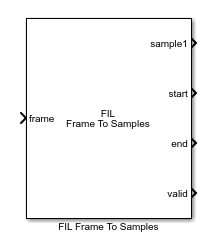# FIL Frame To Samples

Convert frame-based data to sample stream for FPGA-in-the-loop

• Library:
• Wireless HDL Toolbox / I/O Interfaces

•## Description

The FIL Frame To Samples block performs the same frame-to-sample conversion as the Frame To Samples block. It returns output data as vectors of the entire frame of samples. The block returns control signal vectors of the same width as the sample data. This optimization makes more efficient use of the communication link between the FPGA board and your Simulink® simulation when using FPGA-in-the-loop (FIL). To run FPGA-in-the-loop, you must have an HDL Verifier™ license.

When you generate a programming file for a FIL target in Simulink, the tool creates a model to compare the FIL simulation with your Simulink design. For Wireless HDL Toolbox™ designs, the FIL block in that model replicates the sample-streaming interface to send one sample at a time to the FPGA. You can modify the autogenerated model to use the FIL Frame To Samples and FIL Samples To Frame blocks to improve communication bandwidth with the FPGA board by sending one frame at a time. For how to modify the autogenerated model, see FPGA-in-the-Loop.

## Ports

### Input

expand all

Frame of input samples, specified as a column vector. All samples in the vector are considered valid. Each frame must be the same size.

Data Types: `single` | `double` | `int8` | `int16` | `int32` | `uint8` | `uint16` | `uint32` | `Boolean` | `fixed point`

### Output

expand all

Stream of samples, returned as a vector representing an entire frame. The output stream includes idle cycles between samples and between frames as specified in the block parameters.

If you set Output size greater than one, the block shows one port for each output value. In this case, a single sample is represented by N values, such as turbo-encoded samples represented by one systematic value and two parity values. The output data is one vector for each port.

Data Types: `single` | `double` | `int8` | `int16` | `int32` | `uint8` | `uint16` | `uint32` | `Boolean` | `fixed point`

Start of frame, returned as a `Boolean` vector containing one value for each sample in the frame. This signal is 1 (true) for one timestep corresponding to the first valid sample of the frame.

Data Types: `Boolean`

End of frame, returned as a `Boolean` vector containing one value for each sample in the frame. This signal is 1 (true) for one timestep corresponding to the last valid sample of the frame.

Data Types: `Boolean`

Validity of samples, returned as a `Boolean` vector containing one value for each sample in the frame. This signal is 1 (true) on timesteps that correspond to valid samples.

Data Types: `Boolean`

## Parameters

expand all

Number of idle cycles to insert after each sample, specified as a scalar integer. The block returns a zero on each sampleN port for each idle cycle and sets all control signals to 0 (`false`).

Number of idle cycles to insert at the end of each frame, specified as a scalar integer. The block returns a zero on each sampleN port for each idle cycle and sets all control signals to 0 (`false`).

Number of values representing each sample, specified as a positive integer scalar. The block has Output size output sample ports. The control signals apply to all sampleN ports.

For example, you can use this parameter to serialize turbo-encoded samples. In the LTE standard, the turbo code rate is 1/3, so each sample is represented by one systematic value and two parity values: S_n, P1_n, and P2_n. In this case, set Output size to `3`.

Order of output samples relative to input order, when more than one value represents each sample.

For example, for 1/3 turbo-encoded samples, the input frame can be ordered `[S_1 P1_1 P2_1 S_2 P1_2 P2_2]` or ```[S_1 S_2 P1_1 P1_2 P2_1 P2_2]```. In the first case, the output is two vectors, `[S_1 P1_1 P2_1]` and ```[S_2 P1_2 P2_2]```. To achieve the same output in the second case, select Compose output from interleaved input samples.

#### Dependencies

This parameter applies when Output size is greater than one.

## Version History

Introduced in R2017b